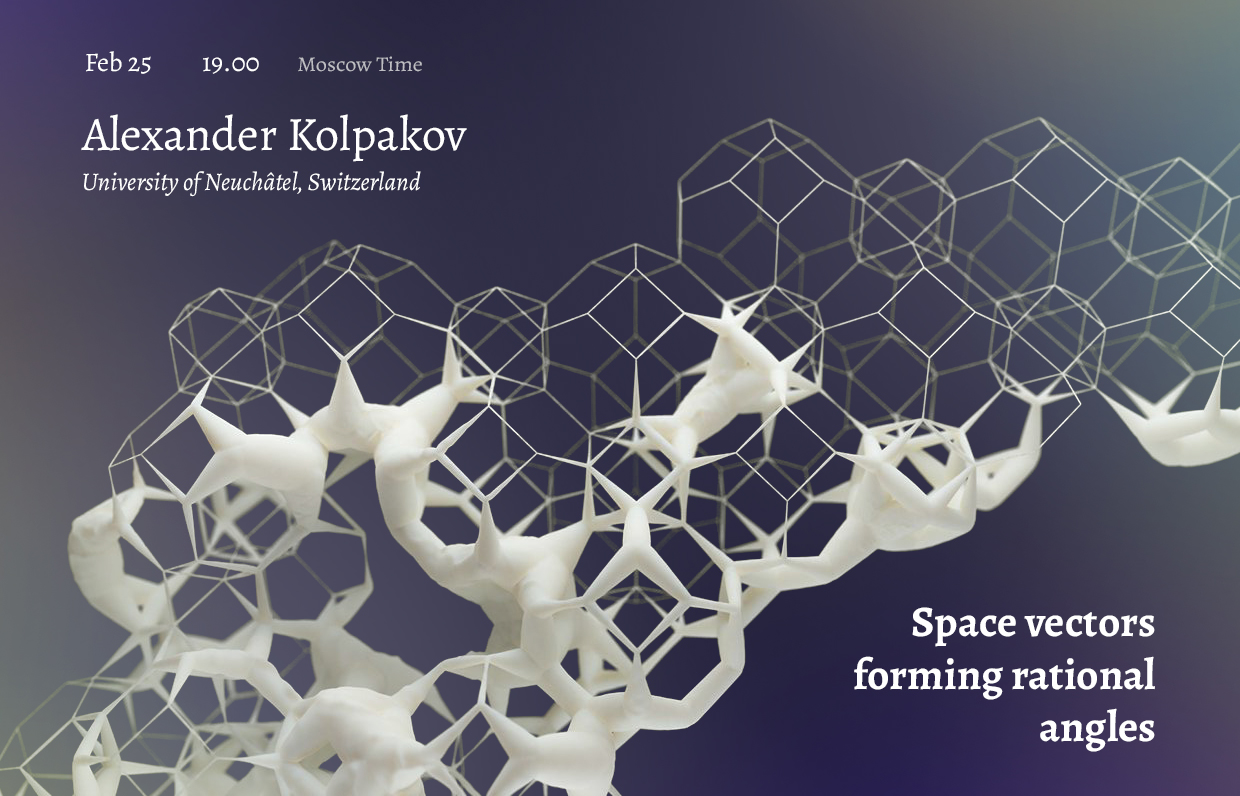# Alexander Kolpakov “Space vectors forming rational angles” | Big SeminarFebruary 25, 2021
19.00 MSK (UTC +3)
Talk on Big Seminar

# Alexander Kolpakov "Space vectors forming rational angles"

Alexander Kolpakov from University of Neuchâtel, Switzerland gave the talk "Space vectors forming rational angles" on the labs' Big Seminar.

The talk will be held in zoom
Meeting ID: 279-059-822
Password: first 6 decimal places of $\pi$ after the decimal point

You can also write to Alexander Polyanskii (alexander.polyanskii@yandex.ru) or to Maksim Zhukovskii (zhukmax@gmail.com) if you want to be added to mailing list.

Abstract:

We classify all sets of nonzero vectors in $\mathbb{R}^3$ such that the angle formed by each pair is a rational multiple of $\pi$. The special case of four-element subsets lets us classify all tetrahedra whose dihedral angles are multiples of $\pi$, solving a 1976 problem of Conway and Jones: there are $2$ one-parameter families and $59$ sporadic tetrahedra, all but three of which are related to either the icosidodecahedron or the $B_3$ root lattice. The proof requires the solution in roots of unity of a $W(D_6)$-symmetric polynomial equation with $105$ monomials (the previous record was only $12$ monomials). This is a joint work with Kiran S. Kedlaya, Bjorn Poonen, and Michael Rubinstein.

## Watch the video:

Everyone is invited to attend. The language of the lecture is English. The event is aimed at master and graduate students, as well as researchers in the field of combinatorics.